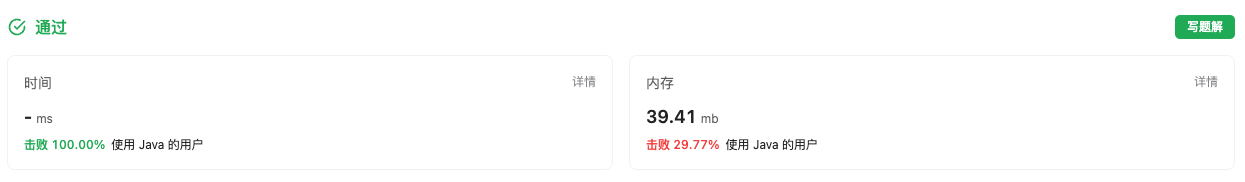#### 88. 合并两个有序数组

###### 来源: 每日一题 2023.08.13

``````输入：nums1 = [1,2,3,0,0,0], m = 3, nums2 = [2,5,6], n = 3

``````

``````输入：nums1 = , m = 1, nums2 = [], n = 0

``````

``````输入：nums1 = , m = 0, nums2 = , n = 1

``````

• `nums1.length == m + n`
• `nums2.length == n`
• `0 <= m, n <= 200`
• `1 <= m + n <= 200`
• `-109 <= nums1[i], nums2[j] <= 109`
``````class Solution {
public void merge(int[] nums1, int m, int[] nums2, int n) {

}
}
``````

#### 分析与题解

• 重拳出击👊🏻 + 双指针 + 模拟法

这个题目要求我们把 `num2` 合并的 `num1` 中, 除了我们需要使用双指针法 + 倒序插入外, 我们需要分析一下几种case情况.

• `m = 0`

• `n = 0`

• `nums1[m] >= num2[n]`

• `nums1[m] < num2[n]`

下面我们就分情况进行讨论.

• `m = 0`

• 我们只需要把 num2[n] 合并到 num1 即可.

``````    nums1[i] = nums2[secondeIndex];
secondeIndex--;
``````
• `n = 0`

• `n = 0` 时, 也就是 num2 中没有了元素, 由于num1是 `非递减数组`, 故这时候直接break 停止循环返回即可.

``````    if(secondeIndex < 0) {
break;
}
``````
• `nums1[m] >= num2[n]`

• `nums1[m] >= num2[n]` 时, 移动 `num1[m]` 位置元素到 `num1[i]` 处即可

``````    nums1[i] = nums1[firstIndex];
firstIndex--;
``````
• `nums1[m] < num2[n]`

• `nums1[m] < num2[n]` 时, 移动 `num2[n]` 位置元素到 `num1[i]` 处即可

``````    nums1[i] = nums2[secondeIndex];
secondeIndex--;
``````

整个题目的题解过程如下所示.

``````class Solution {
public void merge(int[] nums1, int m, int[] nums2, int n) {
int length = nums1.length;
int firstIndex = m - 1;
int secondeIndex = n -1;
for(int i = length - 1; i >= 0; i--) {
if(secondeIndex < 0) {
break;
} else if (firstIndex < 0) {
nums1[i] = nums2[secondeIndex];
secondeIndex--;
} else if(nums1[firstIndex] > nums2[secondeIndex]) {
nums1[i] = nums1[firstIndex];
firstIndex--;
} else {
nums1[i] = nums2[secondeIndex];
secondeIndex--;
}
}
}
}
``````

复杂度分析:

• 时间复杂度: O(n), 一次倒序遍历循环.
• 空间复杂度: O(1), 常量级别的空间复杂度.

结果如下所示.IT界无底坑洞栋主 欢迎加Q骚扰:676758285